# Electronics and Communication Engineering - Exam Questions Papers

41.

Time domain equivalent circuit of the figure is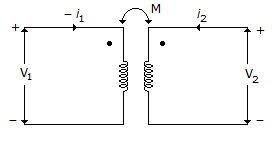A.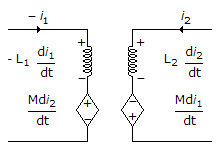B.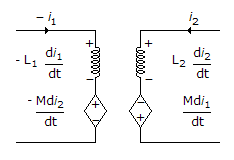C.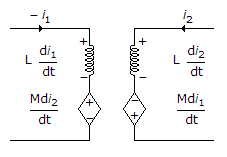D. None of the above

Explanation:

In the left hand loop (- i1) enters the dot end while for the right handed loop i2 enters the dot end.

This will make both the M terms at first of + ve sign but as i1 = - ve hence ultimately the M term of right hand loop involving i1 = - ve.

V1 = - L1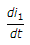+ M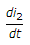V2 = L2- M.

42.

Locus of a point Z satisfying the equation arg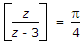is:

 A. Straight line with equation y = x - 3 B. Circle with equation x2 + y2 - 3x + 3y = 0 C. Hyperbola with equation x2 - y2 - 3x - 3y - 2yx = 0 D. None of these

Explanation: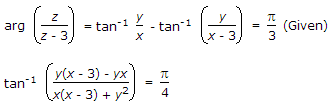Solving this further we will get x2 + y2 - 3x + 3y = 0 which is the equation of circle.

43.

A silicon PN junction with no bias have a doping concentration of
Nd = 2 x 1016 cm-3 and Na = 1016 cm-3. The space charge width is:

 A. 3.345 x 10-3 cm B. 3.345 x 10-4 cm C. 3.345 x 10-5 cm D. 3.345 x 10-6 cm

Explanation: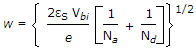w = 3.345 x 10-5 cm.

44.

In 8085, Whenever a signal is received at TRAP terminal its program execution is transferred to a subroutine on address __________ .

 A. 0000 H B. 0024 H C. 002 CH D. provided by the address generation logic

Explanation:

TRAP is considered to be of the highest priority and the address of the subroutine is given by 0024 H.

45.

If (225)Y = (165)8 find value of Y

 A. 7 B. 8 C. 9 D. 11

Explanation:

(225)y => 2Y2 + 2Y + 5 = (165)8 => (117)10

2Y2 + 2Y + 5 = 117

Y2 + Y - 56 = 0

Y = 7 or Y = - 8

Y should be 7.

#### Current Affairs 2022

Interview Questions and Answers